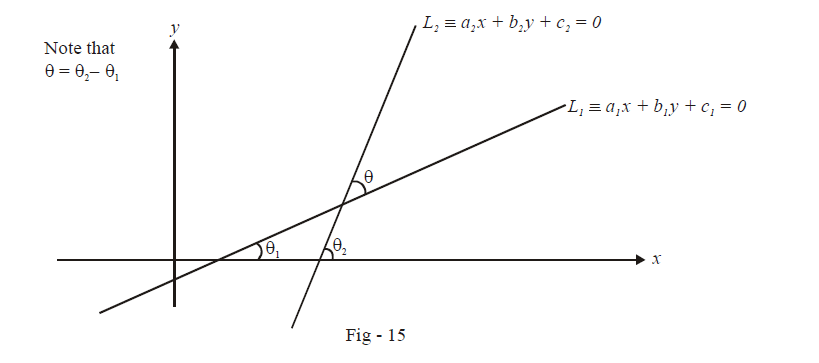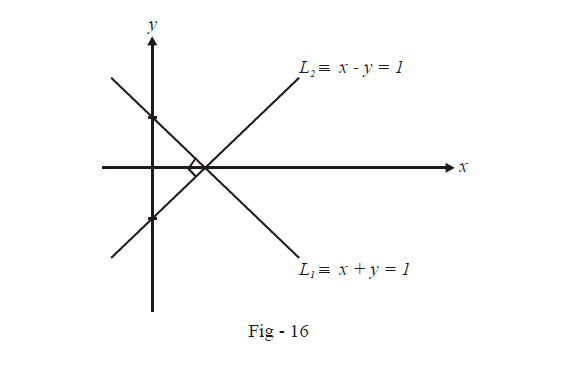# Point and Angle of Intersection of Two Straight Lines

$$\textbf{Art 5 : } \qquad\boxed{{\text{Point of intersection ; Angle of intersection}}}$$

We are given two lines L1 and L2, and we are required to find the point at which they intersect (if they are non-parallel) and the angle at which they are inclined to one another, i.e., the angle of intersection. Evaluating the point of intersection is a simple matter of solving two simultaneous linear equations. Let the equations of the two lines be $${{\text{L}}_{\text{1}}}\equiv {{a}_{1}}x+{{b}_{1}}y+{{c}_{1}}=0\,\,\,\,\,\text{and}\,\,\,{{L}_{2}}\equiv {{a}_{2}}x+{{b}_{2}}y+{{c}_{2}}=0$$ (written in the most general form). Now, let the point of intersection be (x1,  y1). Thus,

\begin{align} & {{a}_{1}}{{x}_{1}}+{{b}_{1}}{{y}_{1}}+{{c}_{1}}=0 \\ & {{a}_{2}}{{x}_{1}}+{{b}_{2}}{{y}_{1}}+{{c}_{2}}=0 \end{align}

This system can be solved to get

\begin{align}\frac{{{x}_{1}}}{{{b}_{1}}{{c}_{2}}-{{b}_{2}}{{c}_{1}}}=\frac{{{y}_{1}}}{{{c}_{1}}{{a}_{2}}-{{c}_{2}}{{a}_{1}}}=\frac{1}{{{a}_{1}}{{b}_{2}}-{{a}_{2}}{{b}_{1}}}\end{align}

From this relation we obtain the point of intersection $$\left( {{x}_{1}},{{y}_{1}} \right)\,\,\text{as}$$

$\boxed{\,\left( {\frac{{{b_1}{c_2} - {b_2}{c_1}}}{{{a_1}{b_2} - {a_2}{b_1}}},\,\frac{{{c_1}{a_2} - {c_2}{c_1}}}{{{a_1}{b_2} - {a_2}{b_1}}}\,} \right)\,}\qquad:\quad \text{Point of intersection}$

To obtain the angle of intersection between these two lines, consider the figure below:The equation of the two lines in slope-intercept form are

\begin{align}& \,\,\,\,\,\,\,\,\,{{y}_{1}}=\left( -\frac{{{a}_{1}}}{{{b}_{1}}} \right)x+\left( \frac{{{c}_{1}}}{{{b}_{1}}} \right)={{m}_{1}}x+\left( \frac{{{c}_{1}}}{{{b}_{1}}} \right)\,\,\text{where}\,\,{{m}_{1}}=-\frac{{{a}_{1}}}{{{b}_{1}}} \\ & \text{and}\,\,{{y}_{2}}=\left( -\frac{{{a}_{2}}}{{{b}_{2}}} \right)x+\left( \frac{{{c}_{2}}}{{{b}_{2}}} \right)={{m}_{2}}x+\left( \frac{{{c}_{2}}}{{{b}_{2}}} \right)\,\,\text{where}\,\,{{m}_{2}}=-\frac{{{a}_{2}}}{{{b}_{2}}} \end{align}

Note in Fig - 15 that $$\theta ={{\theta }_{2}}-{{\theta }_{1}}$$ and thus

\begin{align} & \tan \theta =\tan \left( {{\theta }_{2}}-{{\theta }_{1}} \right) \\\\ & \qquad =\frac{\tan {{\theta }_{2}}-\tan {{\theta }_{1}}}{1+\tan {{\theta }_{1}}\tan {{\theta }_{2}}} \\\\ & \qquad =\frac{{{m}_{2}}-{{m}_{1}}}{1+{{m}_{1}}{{m}_{2}}}\qquad \qquad \qquad ...\text{ }\left( 1 \right) \end{align}

Conventionally, we would be interested only in the acute angle between the two lines and thus we have to have $$\tan\theta$$ as a positive quantity. So in (1) above, if the expression \begin{align}\frac{{{m}_{2}}-{{m}_{1}}}{1+{{m}_{1}}{{m}_{2}}}\end{align} turns out to be negative, this would be the tangent of the obtuse angle between the two lines; thus, to get the acute angle between the two lines, we use the magnitude of this expression.

Therefore, the acute angle $$\theta$$ between the two lines is

$\boxed{\theta = {{\tan }^{ - 1}}\left| {\frac{{{m_2}\,\, - \,{m_1}}}{{1 + {m_1}\,{m_2}}}} \right|\,}\,\,\,\,:\qquad {\mathbf{Acute}}{\text{ }}{\mathbf{angle}}{\text{ }}{\mathbf{between}}{\text{ }}{\mathbf{the}}{\text{ }}{\mathbf{two}}{\text{ }}{\mathbf{lines}}$

From this relation, we can easily deduce the conditions on m1 and m2 such that the two lines L1 and L2 are parallel or perpendicular.

If the lines are parallel,$$\theta = 0$$ so that  $${m_1} = {m_2}$$ which is intuitively obvious since parallel lines must have the same slope. For the two lines to be perpendicular, \begin{align}\theta = \frac{\pi }{2}\end{align} so that $$\cot \theta = 0;$$ this can happen if  $$1 + {m_1}{m_2} = 0\,\quad or\,\quad{m_1}{m_2} = - 1.$$ Thus,

\begin{align}& \boxed{{m_1} = {m_2}:{\text{for}}\,{\text{parallel}}\,{\text{lines}}} \\&\qquad\qquad\qquad and \\&\boxed{{m_1}\,{m_2} = - 1\,\,:\,\,{\text{for}}\,{\text{perpendicular}}\,{\text{lines}}}\end{align}

If the lines L1 and L2 are given in the general form given in the general form  $$ax + by + c = 0,$$ the slope of this line is\begin{align}m = - \frac{a}{b}\end{align} so that the condition for L1 and L2 to be parallel becomes\begin{align} - \frac{{{a_1}}}{{{b_1}}} = - \frac{{{a_2}}}{{{b_2}}}\,\,{\text{or}}\,\,{a_1}{b_2} = {a_2}{b_1}\end{align} and the condition for Land L2 to be perpendicular becomes \begin{align}\frac{{{a_1}{a_2}}}{{{b_1}{b_2}}} = - 1\end{align} or $${a_1}{a_2} + {b_1}{b_2} = 0$$ .

For example, the line $${L_1} \equiv x + y = 1$$ is perpendicular to the line $${L_2} \equiv x - y = 1$$ because the slope of L is –1 while the slope of L2 is 1.As another example, the line $${L_1} \equiv x - 2y + 1 = 0$$ is parallel to the line  $${L_2} \equiv x - 2y - 3 = 0$$ because the slope of both the lines is \begin{align}m = \frac{1}{2}.\end{align}